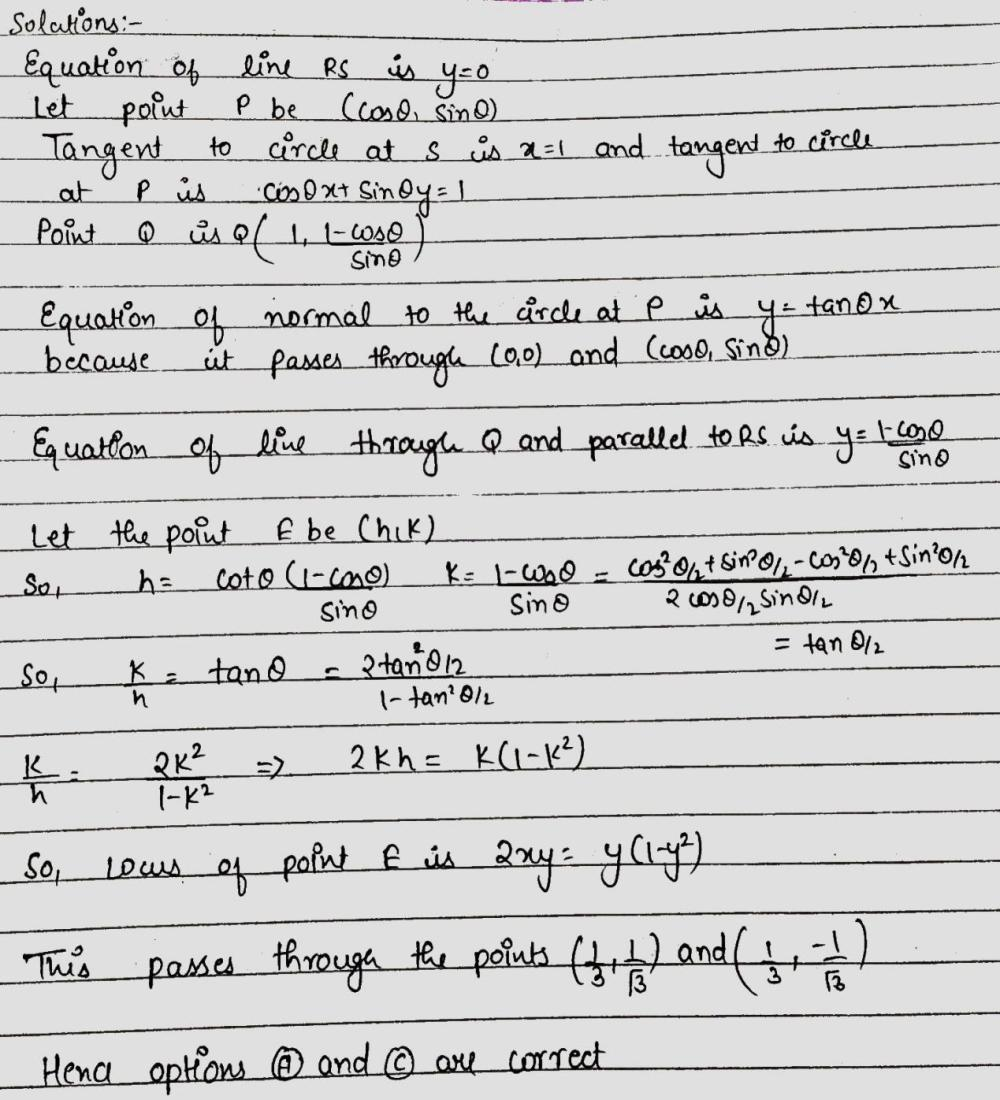Home/Class 11/Maths/

## QuestionMathsClass 11

Let RS be the diameter of the circle $${x}^{2}+{y}^{2}=1,$$ where S is the point $$(1, 0)$$. Let P be a variable point (other than R and S) on the circle and tangents to the circle at S and P meet at the point Q. The normal to the circle at P intersects a line drawn through Q parallel to RS at point E. Then the locus of E passes through the point(s)
A.$$\left(\frac{1}{3}, \frac{1}{\sqrt{3}}\right)$$
B.$$\left(\frac{1}{4}, \frac{1}{2}\right)$$
C.$$\left(\frac{1}{3}, -\frac{1}{\sqrt{3}}\right)$$
D.$$\left(\frac{1}{4}, -\frac{1}{2}\right)$$

4.64.6## Solution